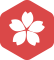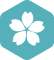### 关于Math.Floor的问题

Delphi > 语言基础/算法/系统设计 [问题点数：100分，结帖人CSDN]2006年5月 Delphi大版内专家分月排行榜第一
2006年4月 Delphi大版内专家分月排行榜第一2006年11月 扩充话题大版内专家分月排行榜第二
2006年9月 扩充话题大版内专家分月排行榜第二
2006年7月 扩充话题大版内专家分月排行榜第二
2006年6月 扩充话题大版内专家分月排行榜第二
2006年5月 扩充话题大版内专家分月排行榜第二
2006年3月 扩充话题大版内专家分月排行榜第二
2006年7月 Delphi大版内专家分月排行榜第二
2006年3月 Delphi大版内专家分月排行榜第二
2005年12月 Delphi大版内专家分月排行榜第二2007年1月 扩充话题大版内专家分月排行榜第三
2006年12月 扩充话题大版内专家分月排行榜第三
2006年10月 扩充话题大版内专家分月排行榜第三
2006年11月 Delphi大版内专家分月排行榜第三
2006年10月 Delphi大版内专家分月排行榜第三
2006年9月 Delphi大版内专家分月排行榜第三2006年2月 Delphi大版内专家分月排行榜第一zhourongbiao

Math.floor的误区

lua中math.floor值偏差的问题

math.floor((1.16-1.0)*100) = 15 math.floor(0.16*100) = 16 考虑到可能是lua中数字都是double类型的原因 所以实验了下以下 math.floor(1.9999999999999999) = 2 math.floor(0.9999999999999999) = 1 又疑惑...

Lua中使用math.floor注意小数问题

local testNum1 = 38.48 print("testNum1 = ", testNum1) ...local testNum3 = math.floor(testNum2) print("testNum3 = ", testNum3) --[[ testNum1 = 38.48 testNum2 = 3848 testNum3 = 3847 -- 问题

Lua中使用math.floor产生bug的问题

Math.round()，Math.ceil()，Math.floor()的区别

1、Math.round() “四舍五入”，该函数返回的是一个四舍五入后的的整数 double d = 3.1415926; double d2 = 18.58; double d3 = -15.23; double d4 = -16.85; long round1 = Math.round(d); //...

Math.Floor（）和Math.Truncate（）之间的区别

.NET中的Math.Floor()和Math.Truncate()什么区别？

Java中Math.floor()和Math.ceil()的区别

Math.ceil()：将小数部分一律向整数部分进位 简单来说，当小数部分为非0值时，进为1.0 Math.ceil(12.1)=13 Math.ceil(12.8)=13 ...Math.floor()：舍去小数，仅取整数部分。 Math.ceil(12.1)=12 Math.ceil(12....

Java中Math.ceil()、Math.floor()、Math.round()的区别

1、Math.ceil() ceil表示“天花板”，向上取整；相当于水平数轴，向左取整 Math.ceil(2.6); //3.0 ceil天花板 水平数轴向左取整 Math.ceil(-2.6); //-2.0 ceil天花板 水平数轴向左取整 ...Math.floor(2....

Math.floor(Math.random() * array.length)

mark js 返回长度范围内的索引：Math.floor(Math.random() * array.length)

Java Math.floor()、Math.ceil()和Math.round()四舍五入的使用及区别

Math.floor()、Math.ceil()和Math.round()都是对浮点数取整（floor和ceil返回不带小数的double，round返回long） floor字面意思为地板，返回的是小于或等于该数值的最大的整数 ceil字面意思是天花板，返回的是大于...

math.floor()函数解析

Math.floor( Math.random() )生成随机整数

Math.floor() method rounds a number DOWNWARDS to the nearest integer, and returns the result. (小于等于 x，且与 x 最接近的整数。) 其实返回值就是该数的整数位： Math.floor(0.666) --&amp;gt; 0 Math....

JS 中Math.ceil().Math.floor()和Math.round()的区别

​ var arg1 = 12.2; var arg2 = 12.5; var arg3 = 12.7; <strong> ceil()：将小数部分一律向整数部分进位<...var c1 = Math.ceil(arg1); var c2 = Math.ceil(arg2); var c3 = Math.ceil(arg3); ...

Math.round()和Math.ceil()和Math.floor()的区别

Math类中提供了三个与取整有关的方法：ceil、floor、round，这些方法的作用与它们的英文名称的含义相对应。 例如，ceil的英文意义是天花板，该方法就表示向上取整，Math.ceil(11.3)的结果为12,Math.ceil(-11.3)的...

math.floor()函数

1.Math.round()：根据“round”的字面意思“附近、周围”，可以猜测该函数是求一个附近的整数，看下面几个例子就明白。 小数点后第一位<5 正数：Math.round(11.46)=11 负数：Math.round(-11.46)=-11 小数点后第一...

Python math.floor()方法 (Python math.floor() method) math.floor() method is a library method of math module, it is used to get the floor value of a given number, it is used to get the floor value of a...

JavaScript Math对象方法 之 Math.abs() Math.ceil() Math.floor();

Math 对象用于执行数学任务。 Math 对象并不像 Date 和 String 那样是对象的类，因此没有构造函数 Math()。 1，Math.abs():方法可返回一个数的绝对值。 语法：Math.abs(x)：如果 x 不是数字返回 NaN,如果 x 为 ...

Math.round()和Math.floor()

Math.round()为四舍五入，但是负数则会舍弃-5。 System.out.println(Math.round(2.6));//3 System.out.println(Math.round(-1.6...Math.floor()为直接去掉小数保留整数，如果参数为正数则小数全舍，参数为负数则全入...

Python3 math.ceil()、math.floor()、//、int()、round()取整问题

Python3 math.ceil()、math.floor()、//、int()、round()取整问题 1. 向上取整：math.ceil()，往较大的方向取 import math math.ceil(2.1) 3 math.ceil(2.8) 3 math.ceil(-2.1) -2 math.ceil(-2.9) -2 2. ...

C#取整函数Math.Round、Math.Ceiling和Math.Floor

1.Math.Round：四舍六入五取偶 引用内容 Math.Round(0.0) //0 Math.Round(0.1) //0 Math.Round(0.2) //0 Math.Round(0.3) //0 Math.Round(0.4) //0 Math.Round(0.5) //0 Math.Round(0.6) //1 Math....

Math.floor(Math.random()*3+1)

Math.random():获取0~1随机数Math.floor() method rounds a number DOWNWARDS to the nearest integer, and returns the result. (小于等于 x，且与 x 最接近的整数。)其实返回值就是该数的整数位：Math.floor(0.666...

Math.round();Math.ceil();Math.floor();取值规则。

C#取整函数Math.Ceiling、Math.Floor简介以及注意使用细节

2、Math.Floor：向下舍位取整。例：Math.Floor(2.6)=2 注意使用细节： Math.Ceiling只支持两种数据类型Decimal和Double，不支持int 类型，Math.Ceiling（Decimal）、Math.Ceiling（Double）。 在定义i...

Android中MathMath.floor()、Math.round()及Math.ceil()等方法的使用

1、Math.floor()先看定义：/** * Returns the double conversion of the most positive (closest to positive * infinity) integer value less than or equal to the argument. * * Special cases: * * <li>

Math的几个方法Math.round()、Math.ceil()、Math.floor()和Math.abs()记录一下

Math.round() 就是数学中的四舍五入，举例： System.out.println("Math.round(1.2)="+Math.round(1.2)); System.out.println("Math.round(1.5)="+Math.round(1.5)); System.out.println("Math.round(1.7)="+...

Math.random和Math.floor取随机数总结

1.Math.random(); //返回一个介于[0,1)之间的随机数 ...但问题在于这样通常会产生小数，这时通过Math.floor();进行解决 2.Math.floor(); will take a decimal number,and round down to the nearest whole nu

JavaScript Math.floor()、Math.random()实现验证码效果

double a = 0.1; double b = 0.50; double c = 0; double d = 0.4; double e = 0.6; double f = 1.3; double g = 1.6; //Math.ceil 表示的是大于某个小数的最小整数的double值

Math.Floor 总是舍去小数

double result1 = Math.Floor(0.3); Console.WriteLine(result1);//result1显示0 double result2 = Math.Floor(0.5); Console.WriteLine(result2);//res...